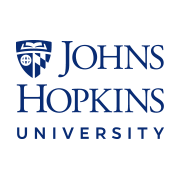## 이 전문 분야 정보

최근 조회 25,493
Fundamental concepts in probability, statistics and linear models are primary building blocks for data science work. Learners aspiring to become biostatisticians and data scientists will benefit from the foundational knowledge being offered in this specialization. It will enable the learner to understand the behind-the-scenes mechanism of key modeling tools in data science, like least squares and linear regression. This specialization starts with Mathematical Statistics bootcamps, specifically concepts and methods used in biostatistics applications. These range from probability, distribution, and likelihood concepts to hypothesis testing and case-control sampling. This specialization also linear models for data science, starting from understanding least squares from a linear algebraic and mathematical perspective, to statistical linear models, including multivariate regression using the R programming language. These courses will give learners a firm foundation in the linear algebraic treatment of regression modeling, which will greatly augment applied data scientists' general understanding of regression models. This specialization requires a fair amount of mathematical sophistication. Basic calculus and linear algebra are required to engage in the content.
학습자 경력 결과
17%
급여 인상 또는 승진하기
공유 가능한 수료증
완료 시 수료증 획득
100% 온라인 강좌
지금 바로 시작해 나만의 일정에 따라 학습을 진행하세요.
유동적 일정
유연한 마감을 설정하고 유지 관리합니다.
고급 단계
완료하는 데 약 5개월 필요
매주 2시간 권장
영어
학습자 경력 결과
17%
급여 인상 또는 승진하기
공유 가능한 수료증
완료 시 수료증 획득
100% 온라인 강좌
지금 바로 시작해 나만의 일정에 따라 학습을 진행하세요.
유동적 일정
유연한 마감을 설정하고 유지 관리합니다.
고급 단계
완료하는 데 약 5개월 필요
매주 2시간 권장
영어

강좌1

4.5
별점
335개의 평가
72개의 리뷰
강좌2

4.4
별점
81개의 평가
17개의 리뷰
강좌3

4.5
별점
144개의 평가
36개의 리뷰
강좌4

## 강좌 4

4.6
별점
65개의 평가
12개의 리뷰

### 제공자:## 자주 묻는 질문

궁금한 점이 더 있으신가요? 학습자 도움말 센터를 방문해 보세요.